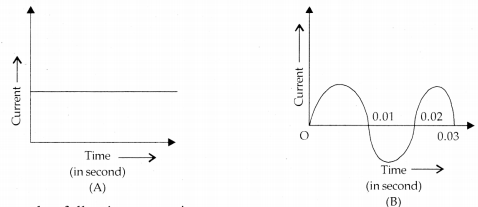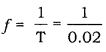# Write one difference between A.C and D.C

Current-time graphs from two different sources are shown in the following diagrams. Now answer the following questions.(1) Name the type of current shown by graph A and graph B.
(ii) Name any one source of the current shown by graph A and graph B.
(iii) What is the frequency of current in case B?
(iv) Write two difference between the current shown by graphs A and B.
OR
Write one difference between A.C and D.C.

1 Like

(i) Graph A represents direct current (D.C). Graph B represents alternating current (A.C).

(ii) A dry cell is the source of current shown by Graph A. An A.C generator is the source of current shown by the graph B.

(iii) From graph B, time period, T = 0.02s. Therefore, frequency of current,= 50Hz.

(iv)

Direct Current Alternating Current
The magnitude of direct current is constant and flows in one direction only. The magnitude of AC changes with time and direction of alternating current reverses periodically.
direct current cannot be used for large scale supply of electricity for household purpose. alternating current is used to run electrical appliance like electric bulb, electric heater, electric iron, refrigerator etc.
The frequency of direct current is zero. Frequency of alternating current in India is 50 Hz.
1 Like